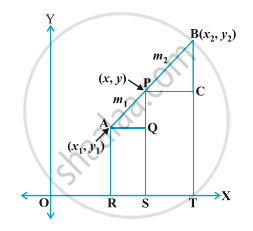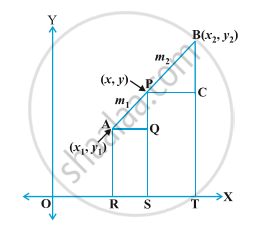# Section Formula

#### definition

Whenever a point divides a line segment into two, three, four or any number of sections in a particular ratio then the formula used there is know as Section Formula.

#### notes

1) Section Formula-Section Formula is derived as follows,Let P be a point (x,y) which divides a line segment AB in the ratio m_1:m_2
"AP"/"PB"= m_1/m_2
Draw AR, PS and BT ⊥ OT
Also, draw AQ ⊥ PS, PC ⊥ BX
AQ=RS (Since AQSR forms a rectangle opposite sides are equal)
RS= OS-OR
Now, after watching carefully, we can see that OS have travelled x units and OR have travelled x_1 units.
RS= (x-x_1)
Therefore, AQ= (x-x_1)
Similarly, PC=ST= (x_2-x)
and QP= PS-QS= (y-y_1)
also, BC= BT-CT= (y_2-y)
Clearly, ΔAQP and ΔPCB are similar triangle.

Therefore, "AP"/"PB"= "AQ"/"PC"= "QP"/"CB"

m_1/m_2= (x-x_1)/(x_2-x) =( y-y_1)/(y_2-y)

m_1/m_2= (x-x_1)/(x_2-x) and m_1/m_2= (y-y_1)/y_2-y

m_1x_2-m_1x = m_2x-m_2x_1 and m_1y_2-m_1y = m_2y-m_2y_1
m_1x_2+m_2x_1= m_1x+m_2x  and m_1y_2+m_2y_1= m_1y+m_2y
m_1x_2+m_2x_1= x(m_1+m_2) and m_1y_2+m_2y_1= y(m_1+m_2)

x= (m_1x_2+m_2x_1)/(m_1+m_2) and y= (m_1y_2+m_2y_1)/(m_1+m_2)

P(x,y)= [(m_1x_2+m_2x_1)/(m_1+m_2), (m_1y_2+m_2y_1)/(m_1+m_2)]

2) Midpoint Formula-Here let's say P is midpoint and divides AB in equal ratio i.e 1:1
Thus, AP:PB= m_1:m_2= 1:1

P(x,y)= [(1x_2+1x_1)/(1+1), (1y_2+1y_1)/(1+1)]

P(x,y)= [(x_2+x_1)/2, (y_2+y_1)/2]

Therefore, midpoint formula= [(x_2+x_1)/2, (y_2+y_1)/2]

If you would like to contribute notes or other learning material, please submit them using the button below.

### Shaalaa.com

Coordinate Geometry part 8 (Sectional formula) [00:13:38]
S
0%